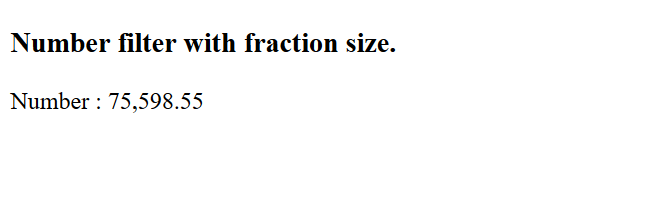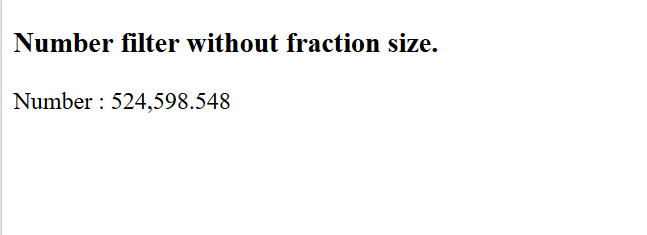# AngularJS | number Filter

AngularJS number filter is used to convert a number into string or text. We can also define a limit to display a number of decimal digits. Number filter rounds off the number to specified decimal digits.

Syntax:

```{{ string| number : fractionSize}}
```

Parameter Values: It contains single parameter value fractionsize which is of type number and used to specify the number of decimals.

Example 1: This example format the number and set it into the fraction with two decimal place.

 ` ` `<``html``> ` `    ``<``head``> ` `        ``<``title``>Number Filter ` `         `  `        ``<``script` `src``= ` `"https://ajax.googleapis.com/ajax/libs/angularjs/1.6.9/angular.min.js"``> ` `        `` ` `    `` ` `     `  `    ``<``body``> ` `  `  `        ``<``div` `ng-app``=``"gfgApp"` `ng-controller``=``"numberCntrl"``> ` `            ``<``h3``>Number filter with fraction size. ` `            ``<``p``>Number : {{ value| number  : 2}} ` `        `` ` `  `  `        ``<``script``> ` `            ``var app = angular.module('gfgApp', []); ` `            ``app.controller('numberCntrl', function(\$scope) { ` `                ``\$scope.value = 75598.548; ` `            ``}); ` `        `` ` `  `  `    `` ` ` `

Output:Example 2: This example format the number and set it into the fraction with three decimal place.

 ` ` `<``html``> ` `    ``<``head``> ` `        ``<``title``>Number Filter ` `         `  `        ``<``script` `src``= ` `"https://ajax.googleapis.com/ajax/libs/angularjs/1.6.9/angular.min.js"``> ` `        `` ` `    `` ` `     `  `    ``<``body``> ` `  `  `        ``<``div` `ng-app``=``"gfgApp"` `ng-controller``=``"numberCntrl"``> ` `            ``<``h3``>Number filter without fraction size. ` `            ``<``p``>Number : {{ value| number}} ` `        `` ` `  `  `        ``<``script``> ` `            ``var app = angular.module('gfgApp', []); ` `            ``app.controller('numberCntrl', function(\$scope) { ` `                ``\$scope.value = 524598.54812; ` `            ``}); ` `        `` ` `  `  `    `` ` ` `

Output:My Personal Notes arrow_drop_upCheck out this Author's contributed articles.

If you like GeeksforGeeks and would like to contribute, you can also write an article using contribute.geeksforgeeks.org or mail your article to contribute@geeksforgeeks.org. See your article appearing on the GeeksforGeeks main page and help other Geeks.

Please Improve this article if you find anything incorrect by clicking on the "Improve Article" button below.

Article Tags :

Be the First to upvote.

Please write to us at contribute@geeksforgeeks.org to report any issue with the above content.